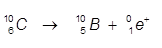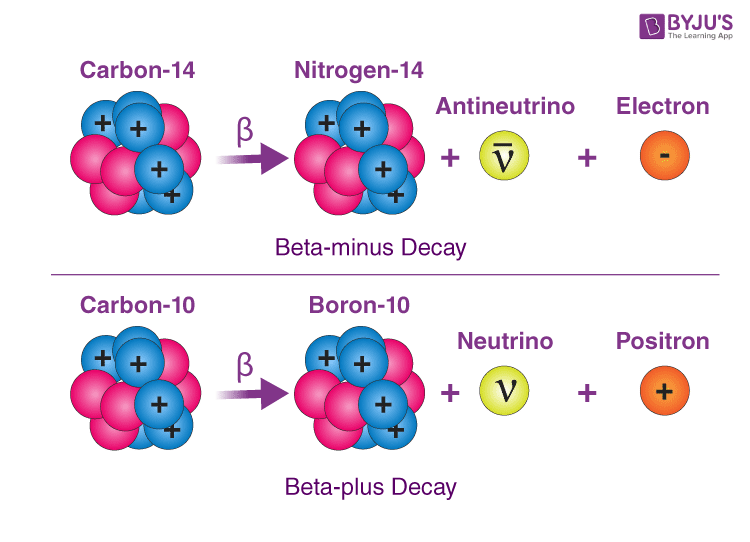# Beta Decay

When dealing with nuclear reactions, several different types of reactions can occur, one of them being beta decay, which is when a neutron decomposes into a proton and an electron. Let us familiarize ourselves with beta decay and its process by reading the article.

## What is Beta Decay?

Beta Decay is a type of radioactive decay in which a proton is transformed into a neutron or vice versa inside the nucleus of the radioactive sample. Processes like beta decay and alpha decay allow the nucleus of the radioactive sample to get as close as possible to the optimum neutron/ proton ratio. While doing so, the nucleus emits a beta particle which can either be an electron or positron. Remember that there either a proton can turn to a neutron or neutron to a proton. Electron and the positron are generated to obey the law of conservation of charge. Beta-decay occurs via the weak interaction.

### What happens in Beta Decay?

Beta decay is a radioactive decay in which a beta ray is emitted from an atomic nucleus. During beta decay, the proton in the nucleus is transformed into a neutron and vice versa. If a proton is converted to neutron, it is known as β+ decay. Similarly, if a neutron is converted to a proton, it is known as β- decay. Due to the change in the nucleus, a beta particle is emitted. The beta particle is a high-speed electron when it is a β- decay and a positron when it is a β+ decay. Beta particles are used to treat health conditions such as eye and bone cancer and are also used as tracers.

### Beta Decay Example

One of the examples of beta decay is the β- decay of the carbon atom. Here, a neutron of carbon is converted into a proton, and the emitted beta particle is an electron. Similarly, the β+ decay of carbon-10 can be represented by an equation as follows:Here, the proton of the carbon atom is converted into a neutron, and the emitted beta particle is a positron.

## Types of Beta Decay

There are two beta decay types: beta minus (&beta) and beta plus (&beta+).

### Beta-Minus Decay

• In beta minus, a neutron is transformed to yield a proton, causing an increase in the atom’s atomic number. The neutron is neutral, but the proton is positive.
• To maintain the conservation of charge, the nucleus in the process also produces an electron and an antineutrino.
• Antineutrino is the antimatter counterpart of neutrino. Both of these are neutral particles with negligible mass. They interact with matter very weakly and can even pass through the entire earth without being disturbed.
• In beta minus decay, the change in atomic configuration is:

$$_{Z}^{A}\textrm{X} \rightarrow _{Z+1}^{A}\textrm{Y} + e^{-} + \bar{\nu }$$

$$N$$ = $$p + e^{-} + v^-$$

Examples of beta minus decay include the decay of $$^{14}C$$ into $$^{14}N$$ and it usually occurs in neutron rich nuclei.

### Beta-Plus Decay

• In beta plus decay, the proton disintegrates to yield a neutron causing a decrease in the atomic number of the radioactive sample. The nucleus experiences a loss of proton but gains a neutron.
• Again, conservation of charge is important. The beta plus decay in order to obey the conservation law also yields a positron and a neutrino.
• A positron is the antimatter equivalent of an electron; the same in all aspects except that a positron has a positive charge.
• A Neutrino’s behaviour is the same as the antineutrinos. Expressed in the equation it is:

$$_{Z}^{A}\textrm{X} \rightarrow _{Z-1}^{A}\textrm{Y} + e^{+} + {\nu }$$

$$P$$ = $$n + e^+ + v$$

Beta plus decay can happen only if the daughter nucleus is more stable than the mother nucleus. This difference goes into the conversion of a proton into a neutron, a positron and a neutrino. There is no increase in mass number because a proton and a neutron have the same mass.

The below image depicts the example of beta minus (β) decay and beta plus (β+) decay.## Fermi’s Theory of Beta Decay

Fermi’s theory of beta decay or Fermi’s interaction illustrates beta decay by Enrico Fermi in 1933. Enrico Fermi created the world’s first nuclear reactor.

He proposed that four fermions directly interact with one another at one vertex. This interaction explains the beta decay by direct coupling of a neutron with an electron, a neutrino (later determined to be an antineutrino), and a proton.

 Similar Articles:

## Frequently Asked Questions – FAQs

### What is beta decay?

Beta Decay is a type of radioactive decay in which a proton is transformed into a neutron or vice versa inside the nucleus of the radioactive sample.

### What is a beta decay example?

One of the examples of beta decay is the β- decay of carbon atoms.

### What are beta particles?

The beta particle is a high-speed electron when it is a β- decay and a positron when it is a β+ decay. Here, a neutron of carbon is converted into a proton and the emitted beta particle is an electron.

### What are the uses of beta decay?

Beta particles are used to treat health conditions such as eye and bone cancer and are also used as tracers.

### What happens in beta-plus decay?

In beta plus decay, the proton disintegrates to yield a neutron causing a decrease in the atomic number of the radioactive sample.

Among the various proposed models over the years, the Quantum Mechanical Model seems to fit all properties best. Why? Watch the video to learn more.Stay tuned to BYJU’S and Fall in Love with Learning!